Next: The Program Potential Up: Writing Down the Equations Previous: Writing Down the Equations

### Variable Rescalings

The first thing you will need to decide about your model is how to rescale the variables. In general they are rescaled as(4.3)

You may set the variables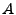,, andto whatever you wish. The variable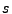must be set to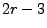for the program to work correctly, so this setting is done automatically by the program. If you have in mind a particular set of rescalings that you think most simplifies your model then you can simply define these three variables appropriately. In general, however, there are a set of default values that should work well for any model where the dominant term is polynomial in the fields (or can be approximated as such). These default rescalings are derived and explained in section 6.1.2. Here we simply quote the results. Assuming the dominant term in your potential is of the form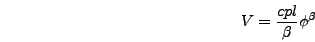(4.4)

whereis one of the fields in your problem, the default values for the rescaling variables are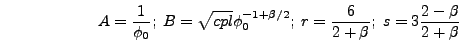(4.5)

whereis the initial value of the field. It is often most convenient to choose this value such that the derivatives of the homogeneous fields vanish initially, but this is not necessary.

For the TWOFLDLAMBDA model the dominant term is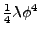so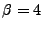and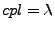. This means that(4.6)

From equation (4.5) the time derivative ofin program variables is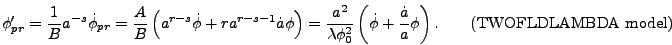(4.7)

Before running this model on the lattice we solved the ODE for the evolution of a homogeneous fieldwith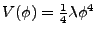near the end of inflation and determined that the expression above equals zero at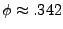. (Recall that all numbers are given here in Planck units.) So we set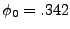and for initial conditions set the homogeneous component of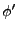to zero.Next: The Program Potential Up: Writing Down the Equations Previous: Writing Down the Equations